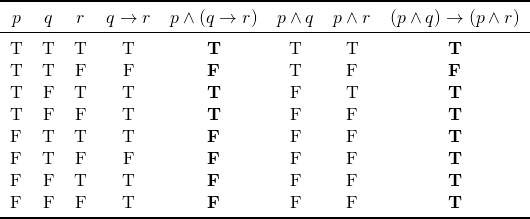# Is there a distributive rule for the implication?

We know that we have distributive rules that handle the disjunction and the conjunction, such as the following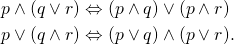But, does there exist a rule, for example, that would handle distributivity of the conjunction with the implication? In other words,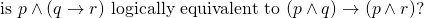At least, the above case, the answer is no. We can see this by constructing a truth table like the one below; the truth values of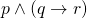are not the same as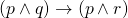.  Hence,andare not logically equivalent. Therefore, it’s not a rule that we can apply in our proofs.